# Cuboids, Rectangular Prisms and Cubes

Go to Surface Area or Volume.A cuboid is a box-shaped object.

It has six flat faces and all angles are right angles.

And all of its faces are rectangles.

It is also a prism because it has the same cross-section along a length. In fact it is a rectangular prism.

## Examples of Cuboids

Cuboids are very common in our world, from boxes to buildings we see them everywhere. We can even fit them inside other cuboids!A buildingA box with a
slot as a handle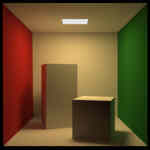Cuboids in a
cuboid room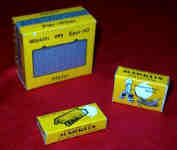Boxes for model trainsNow that's just silly!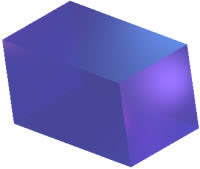## Square Prism

When at least two of the lengths are equal it can also be called a square prism.

(Note: we can still call it a rectangular prism if we want!)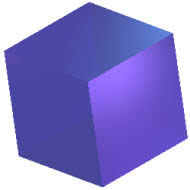## Cube

When all three lengths are equal it is called a cube (or hexahedron)
and each face is a square.

A cube is still a prism.

And a cube is one of the Platonic Solids.

So:
• A cube is just a special case of a square prism, and
• A square prism is just a special case of a rectangular prism, and
• They are all cuboids!

Note: The name "cuboid" comes from "cube" and -oid (which means "similar to, or resembling") and so says "it is like a cube".

Another use of -oid is when we talk about the Earth being a spheroid (not exactly a sphere, but close).

## Surface Area

The surface area is found using the formula:

Area = 2 × Width × Length + 2 × Length × Height + 2 × Width × Height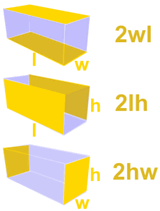Which can be shortened to:

A = 2wl + 2lh + 2hw

### Example: Find the surface area of this cuboidA = 2wl + 2lh + 2hw = 2×4×10 + 2×10×5 + 2×5×4 = 80 + 100 + 40 = 220

## Volume

The volume of a cuboid is found using the formula:

Volume = Length × Width ×  Height

Which can be shortened to:

V = l × w × h

Or more simply:

V = lwh

### Example: Find the volume of this cuboidV = lwh    = 10×4×5    = 200